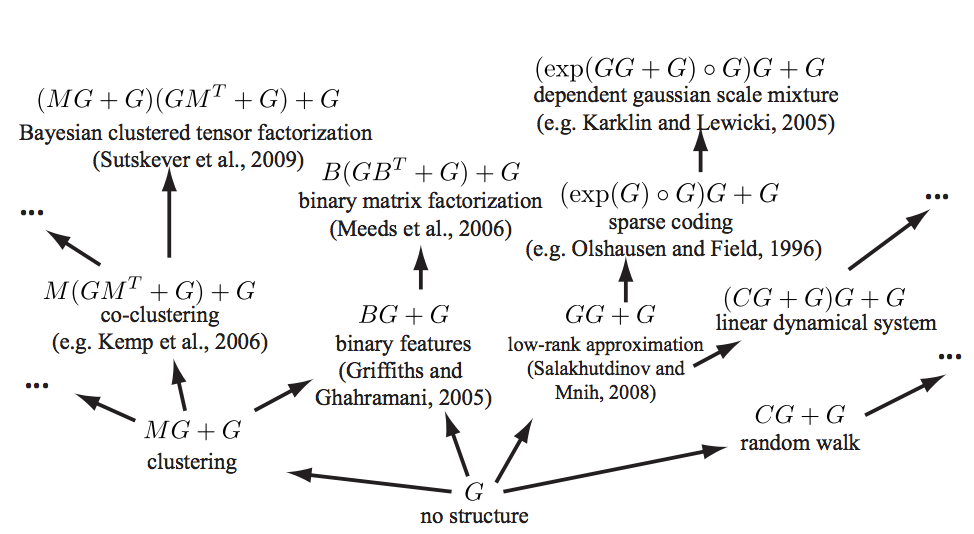# Combining kernelsA sum or product (or outer sum, or tensor product) of Mercer kernels is still a kernel. For other operations YMMV.

## Operations preserving positivity

For example, in the case of Gaussian processes, suppose that, independently,

\begin{aligned} f_{1} &\sim \mathcal{GP}\left(\mu_{1}, k_{1}\right)\\ f_{2} &\sim \mathcal{GP}\left(\mu_{2}, k_{2}\right) \end{aligned} then

$f_{1}+f_{2} \sim \mathcal{GP} \left(\mu_{1}+\mu_{2}, k_{1}+k_{2}\right)$ so $$k_{1}+k_{2}$$ is also a kernel.

More generally, if $$k_{1}$$ and $$k_{2}$$ are two kernels, and $$c_{1}$$, and $$c_{2}$$ are two positive real numbers, then:

$K(x, x')=c_{1} k_{1}(x, x')+c_{2} k_{2}(x, x')$ is again a kernel. What with the multiplication as well, we note that all polynomials of kernels where the coefficients are positive are in turn kernels.

Note that the additivity in terms of kernels is not the same as additivity in terms of induced feature spaces. The induced feature map of $$k_{1}+k_{2}$$ is their concatenation rather than their sum. Suppose $$\phi_{1}(x)$$ gives us the feature map of $$k_{1}$$ for $$x$$ and likewise $$\phi_{2}(x)$$.

\begin{aligned} k_{1}(x, x') &=\phi_{1}(x)^{\top} \phi_{1}(x') \\ k_{2}(x, x') &=\phi_{2}(x)^{\top} \phi_{2}(x')\\ k_{1}(x, x')+k_{2}(x, x') &=\phi_{1}(x)^{\top} \phi_{1}(x')+\phi_{2}(x)^{\top} \phi_{2}(x')\\ &=\left[\begin{array}{c}{\phi_{1}(x)} \\ {\phi_{2}(x)}\end{array}\right]^{\top} \left[\begin{array}{c}{\phi_{1}(x')} \\ {\phi_{2}(x')}\end{array}\right] \end{aligned}

If $$k:\mathcal{Y}\times\mathcal{Y}\to\mathbb{R}$$ is a kernel and $$\psi: \mathcal{X}\to\mathcal{Y}$$ this is also a kernel

\begin{aligned} k_{\psi}:&\mathcal{X}\times\mathcal{X}\to\mathbb{R}\\ & (x,x')\mapsto k_{y}(\psi(x), \psi(x')) \end{aligned}

which apparently is now called a deep kernel. if $$k$$ is stationary and $$\psi$$ is invertible then this is a stationary reducible kernel.

Also if $$A$$ is a positive definite operator, then of course it defines a kernel $$k_A(x,x'):=x^{\top}Ax'$$

uses the properties of covariance to construct some other nifty ones:

Let $$h:\mathcal{X}\to\mathbb{R}^{+}$$ have minimum at 0. Then, using the identity for RVs

$\mathop{\textrm{Cov}}\left(Y_{1}, Y_{2}\right)=\left[\mathop{\textrm{Var}}\left(Y_{1}+Y_{2}\right)-\mathop{\textrm{Var}}\left(Y_{1}-Y_{2}\right)\right] / 4$

we find that the following is a kernel

$K(x, x')=\frac{1}{4}[h(x+x')-h(x-x')]$

All these to various cunning combination strategies, which I may return to discuss. 🏗 Some of them are in the references. For example position their work in the wider field:

There is a large body of work attempting to construct a rich kernel through a weighted sum of base kernels . While these approaches find the optimal solution in polynomial time, speed comes at a cost: the component kernels, as well as their hyperparameters, must be specified in advance […]

Hinton and Salakhutdinov (2008) use a deep neural network to learn an embedding; this is a flexible approach to kernel learning but relies upon finding structure in the input density, $$p(x)$$. Instead we focus on domains where most of the interesting structure is in $$f(x)$$.

Wilson and Adams (2013) derive kernels of the form $$\operatorname{SE} \times \cos(x − x_0$$), forming a basis for stationary kernels. These kernels share similarities with $$\operatorname{SE} \times \operatorname{Per}$$ but can express negative prior correlation, and could usefully be included in our grammar.

See for a mind-melting compositional matrix factorization diagram, constructing a search over hierarchical kernel decompositions.“Exploiting compositionality to explore a large space of model structures” indeed.

Examples of existing machine learning models which fall under our framework. Arrows represent models reachable using a single production rule. Only a small fraction of the 2496 models reachable within 3 steps are shown, and not all possible arrows are shown.

Here is another list of kernel combinations that preserve positive-definiteness, but I have tragically lost the source for it (it is one of the papers here).

\begin{aligned} K(x, y) &=K_{1}(x, y)+K_{2}(x, y) \\ K(x, y) &=c K_{1}(x, y)+K_{2}(x, y) \text { for } c \in \mathbb{R}_{+} \\ K(x, y) &=K_{1}(x, y)+c \text { for } c \in \mathbb{R}_{+} \\ K(x, y) &=K_{1}(x, y) K_{2}(x, y) \\ K(x, y) &=f(x) f(y) \text { for } f: \mathcal{X} \to \mathbb{R} \\ K(x, y) &=\left(K_{1}(x, y)+c\right)^{d} \text { for } \theta_{1} \in \mathbb{R}_{+} \text {and } d \in \mathbb{N} \\ K(x, y) &=\exp \left(K_{1}(x, y) / \sigma^{2}\right) \text { for } \sigma \in \mathbb{R} \\ K(x, y) &=\exp \left(-\left(K_{1}(x, x)-2 K_{1}(x, y)+K_{1}(y, y)\right) / 2 \sigma^{2}\right) \\ K(x, y) &=K_{1}(x, y) / \sqrt{K_{1}(x, x) K_{1}(y, y)} \end{aligned}

## Applying kernels over different axes

I silently assumed it because it seems obvious sometimes; but then sometimes I forget this trick, so maybe it is not obvious:

For multi-dimensional inputs we can apply kernels over any subset of the axes, and thence different kernels ov er different subsets of the axes.

## Locally stationary kernels

defines these as kernels that have a particular structure, specifically, a kernel that can be factored into a stationary kernel $$K_2$$ and a non negative function $$K_1$$ in the following way:

$K(\mathbf{s}, \mathbf{t})=K_{1}\left(\frac{\mathbf{s}+\mathbf{t}}{2}\right) K_{2}(\mathbf{s}-\mathbf{t})$

Global structure then depends on the mean location $$\frac{\mathbf{s}+\mathbf{t}}{2}$$. describes some nifty spectral properties of these kernels.

Other constructions might vie for the title of “locally stationary”. To check. 🏗

## Stationary reducible kernels

See kernel warping.

## Other nonstationary kernels

### No comments yet. Why not leave one?

GitHub-flavored Markdown & a sane subset of HTML is supported.